Programs for Mechanical Waves (with Sound)
Below are all the programs that might be used with this topic. Click on "See Resources" to see if there are any student directions, lab sheets or other materials that have been created for this program.
Reflecting Waves

LabInterfering Waves

Lab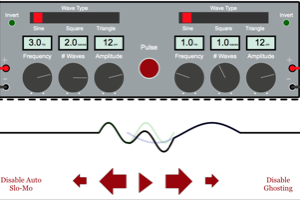Standing Waves

Lab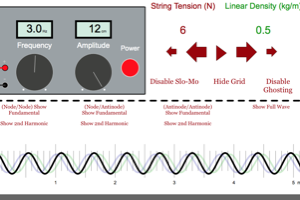Resonance Tubes

Lab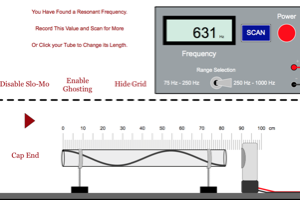Distance to Destruction

LabSpeed of Wave on String

Lab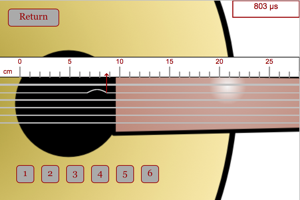Speed of Sound

Lab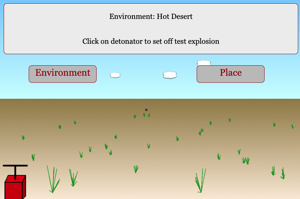Wavelength

LabIntensity

Lab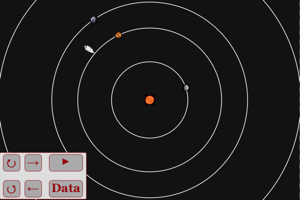Beats

Lab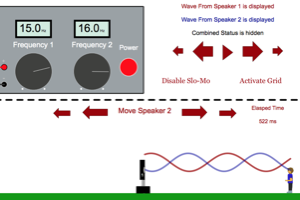Doppler Effect

LabDiffraction of Light

LabFrequency for Standing Wave on a String

HomeworkWave Properties from Graph 1

Homework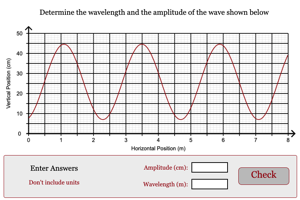Height of Firework

HomeworkWave Properties from Graph 2

Homework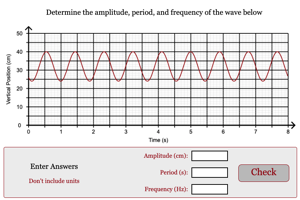Wave Equations

HomeworkSound Wave Problem

HomeworkSound Intensity Problem

Homework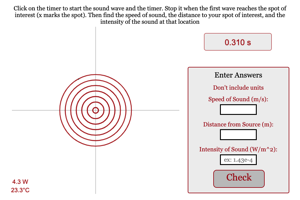Frequency for Standing Wave on a String

HomeworkWave Properties from Graph 3

Homework